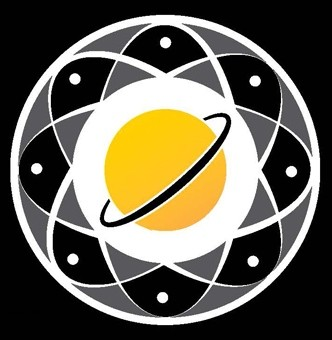## work-and-energy-mcqs# Work and Energy MCQs:

Work is defined as the force exerted by an object on another object multiplied by the distance over which the force is exerted. Energy is the ability of a system to do work.

Work and energy are intimately related energy must be present for work to be done. There are two types of energy - potential and kinetic. Potential energy is stored energy while kinetic energy is energy in motion.

Work and energy MCQs are essential physics topics and which types cover work and energy? Are work and energy MCQs beneficial for students? Work and energy MCQs are essential in physics allowing students to test their understanding of these concepts.

Two types of MCQs cover this topic - conceptual questions and calculations-based questions. Conceptual questions test a students understanding of the key concepts.

In contrast calculation-based questions require students to apply these concepts to solve problems. Both types of questions are beneficial for students as they help to consolidate what has been learnt and ensure that students have a thorough understanding of the material.

There are many different types of MCQs but most of them can be classified into two main categories: conceptual and numerical.

Conceptual MCQs test your understanding of the concepts behind work and energy. They will often ask you to identify the correct definition of work or energy or to explain how one concept relates to another.

Numerical MCQs test your ability to apply the equations that govern work and energy. They will often ask you to solve problems.

Taking these MCQs can help students better understand the concepts of work and energy. Work and energy MCQs benefit students because they can help them learn more about these critical concepts.

In addition taking these MCQs can also help students prepare for exams.

Work and energy are essential topics in physics because they allow us to understand and predict the behaviour of systems.

By understanding how work and energy are related we can develop models that describe object motion and energy transfer between them.

There are two types of work and energy MCQs: multiple-choice and free-response. In multiple-choice questions students are given a question and several possible answers.

They must select the correct answer from the choices provided. Free-response questions require students to write their own answers to a question. Both types of MCQs benefit students because they allow them to test their knowledge and understanding of work and energy.

Work and Energy Multiple-choice questions help students identify the key concepts they need to know. In contrast free-response questions allow them to apply what they have learned to new situations.

Name Category Option
work-and-energy-mcqs Physics_Mcqs